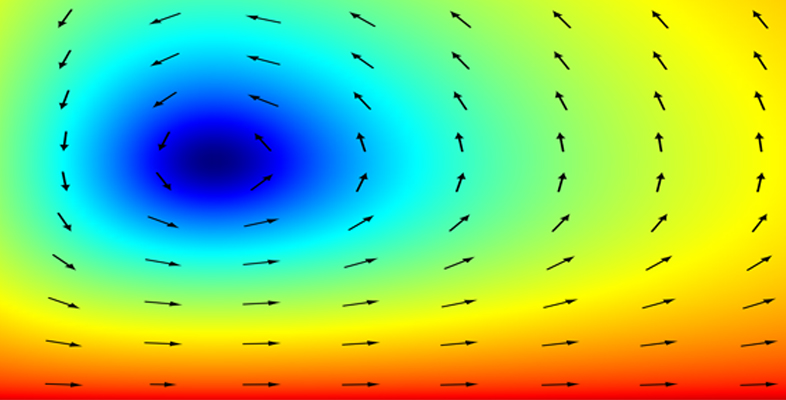Dynamics

Start this free course now. Just create an account and sign in. Enrol on the course to track your learning.

Free course

# Conclusion

Following completion of this free OpenLearn course, Dynamics, as well as being able to ‘apply Newton’s laws of motion by translating them into useful mathematical equations’ you should also find that your skills in problem solving are improving.

You should now be able to:

• understand and use basic terms for the description of the motion of particles, vector functions and the fundamental laws of Newtonian mechanics
• solve mechanics problems in one dimension that involve one or more of the forces of gravity, friction and air resistance
• understand the concept of terminal speed, and use it in solving mechanics problems in one dimension
• apply Newton’s second law in vector form to problems in more than one dimension
• solve problems relating to the motion of a projectile in the absence of air resistance.

This free OpenLearn course is an extract from the Open University course MST210 Mathematical methods, models and modelling [Tip: hold Ctrl and click a link to open it in a new tab. (Hide tip)] .

If you feel you are ready to move on in your study of applied mathematics but don’t have time to study a full Open University course at this time, you might like to study the free OpenLearn course Second-order differential equations, an adapted extract from the Open University course MST224 Mathematical methods.

Alternatively, if you are interested in pure mathematics, you might like to try the free OpenLearn courses Introduction to group theory, Introduction to linear equations and matrices and Introduction to analysis. If you are interested in statistics, you might like to try the free OpenLearn courses Modelling and estimation, Medical statistics and Bayesian statistics.

MST210_1